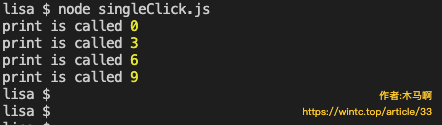## 一、闭包的概念和特性

``````function makeFab () {
let last = 1, current = 1
return function inner() {
[current, last] = [current + last, current]
return last
}
}

let fab = makeFab()
console.log(fab()) // 1
console.log(fab()) // 2
console.log(fab()) // 3
console.log(fab()) // 5``````

MDN中给出的闭包的定义是：函数与对其状态即词法环境的引用共同构成闭包。这里的“词法环境的引用”，可以简单理解为“引用了函数外部的一些变量”，例如上述例子中每次调用makeFab都会创建并返回inner函数，引用了last和current两个变量。

## 二、闭包——函数式编程之魂

Javascript和python这两门动态语言都强调一个概念：万物皆对象。自然，函数也是对象。

``````function confirm (confirmText, confirmCallback, cancelCallback) {
// 插入提示框DOM，包含提示语句、确认按钮、取消按钮
// 添加确认按钮点击事件，事件函数中做dom清理工作并调用confirmCallback
// 添加取消按钮点击事件，事件函数中做dom清理工作并调用cancelCallback
}``````

``````function removeItem (id) {
confirm('确认删除吗？', () => {
// 用户点击确认, 发送远程ajax请求
api.removeItem(id).then(xxx)
}, () => {
// 用户点击取消，
console.log('取消删除')
})
}``````

## 三、闭包的一些例子

#### 1. 防抖、节流函数

``````function debounce (func, time) {
let timer = 0
return function (...args) {
timer && clearTimeout(timer)
timer = setTimeout(() => {
timer = 0
func.apply(this, args)
}, time)
}
}

input.onkeypress = debounce(function () {
console.log(input.value) // 事件处理逻辑
}, 500)``````

debounce函数每次调用时，都会创建一个新的闭包函数，该函数保留了对事件逻辑处理函数func以及防抖时间间隔time以及定时器标志timer的引用。类似的还有节流函数：

``````function throttle(func, time) {
let timer = 0 // 定时器标记相当于一个锁标志
return function (...args) {
if (timer) return
func.apply(this, args)
timer = setTimeout(() => timer = 0, time)
}
}``````

#### 2. 优雅解决按钮多次连续点击问题

``````let clickButton = (function () {
let lock = false
return function (postParams) {
if (lock) return
lock = true
// 使用axios发送请求
axios.post('urlxxx', postParams).then(
// 表单提交成功
).catch(error => {
// 表单提交出错
console.log(error)
}).finally(() => {
// 不管成功失败 都解锁
lock = false
})
}
})()

``````function singleClick(func, manuDone = false) {
let lock = false
return function (...args) {
if (lock) return
lock = true
let done = () => lock = false
if (manuDone) return func.call(this, ...args, done)
let promise = func.call(this, ...args)
promise ? promise.finally(done) : done()
return promise
}
}``````

``````let clickButton = singleClick(function (postParams) {
if (!checkForm()) return
return axios.post('urlxxx', postParams).then(
// 表单提交成功
).catch(error => {
// 表单提交出错
console.log(error)
})
})

``````let print = singleClick(function (i, done) {
console.log('print is called', i)
setTimeout(done, 2000)
}, true)

function test () {
for (let i = 0; i < 10; i++) {
setTimeout(() => {
print(i)
}, i * 1000)
}
}
``````

print函数使用singleClick装饰，每次调用2秒后重置lock变量，测试每秒调用一次print函数，执行代码输出如下图：可以看到，其中一些调用没有打印结果，这正是我们想要的结果！singleClick装饰器比每次设置lock变量要方便许多，这里singleClick函数的返回值，以及其中的done函数，都是一个闭包。

#### 3. 闭包模拟私有方法或者变量

“封装”是面向对象的特性之一，所谓“封装”，即一个对象对外隐藏了其内部的一些属性或者方法的实现细节，外界仅能通过暴露的接口操作该对象。JS是比较“自由”的语言，所以并没有类似C++语言那样提供私有变量或成员函数的定义方式，不过利用闭包，却可以很好地模拟这个特性。

``````function makePlayer () {
let exp = 0 // 经验值
return {
getExp () {
return exp
},
changeExp (delta, sReason = '') {
// log(xxx),记录变动日志
exp += delta
}
}
}

let p = makePlayer()
console.log(p.getExp()) // 0
p.changeExp(2000)
console.log(p.getExp()) // 2000``````

¥赞赏